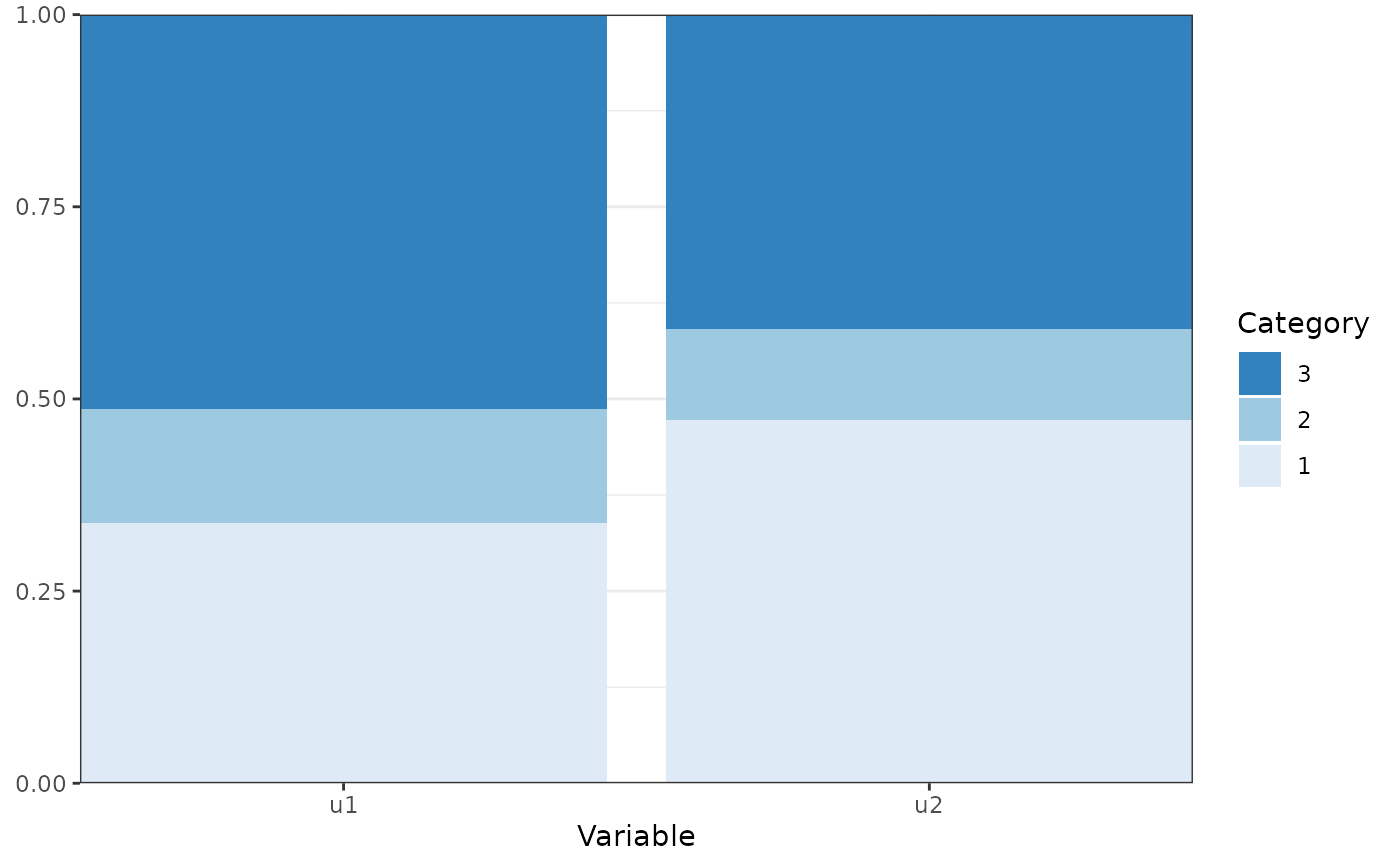Creates a bar chart of categorical variable probabilities with bars reflecting the probability of category membership for each category of the observed variable.

plot_prob(
x,
variables = NULL,
bars = c("Variable", "group", "class"),
facet = c("group", "class", "Variable"),
bw = FALSE,
...
)

## Arguments

x

An object for which a method exists

variables

A character vectors with the names of the variables to be plotted (optional).

bars

Atomic character, indicating what separate bars represent. One of c("Variable", "group", "class").

facet

Atomic character, indicating what separate facets represent. One of c("group", "class", "Variable").

bw

Logical. Should the plot be black and white (for print), or color?

...

Arguments passed to and from other functions.

## Value

An object of class 'ggplot'.

## Author

Caspar J. van Lissa

## Examples

df_plot <- data.frame(Variable = rep(c("u1", "u2"), each = 3),
Category = rep(1:3, 2),
Probability = c(0.3381302605812, 0.148395173612088, 0.513474565806711,
0.472337708760608, 0.118484201496432, 0.40917808974296))
plot_prob(df_plot)